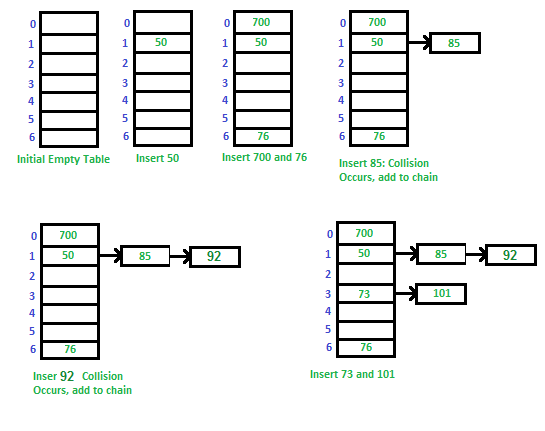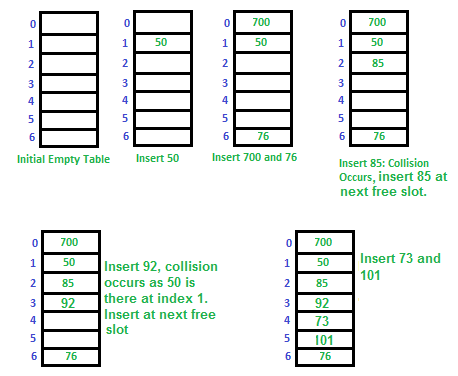# Lesson 4 - Hashing

To introduce the rationale behind hashing, image a hypothetical system for storing employee records keyed using phone numbers. The system should allow the following types of operations to be performed efficiently:
• Insert a phone number and corresponding information.
• Search a phone number and fetch the information.
• Delete a phone number and related information.

Using the techniques we have covered so far, we can think about using the following types of data structures:
• Array of phone numbers and records.
• Linked List of phone numbers and records.
• Balanced binary search tree with phone numbers as keys.
• Direct Access Table.

Arrays and linked lists can be searched in a linear fashion, which can be costly in practice. If we use arrays and keep the data sorted, then a phone number can be searched in O(logn) time using binary Search, but insert and delete operations become costly as we have to constantly maintain the sorted order. This can be mitigated to some extent by sorting lazily when a search operation is requested.

With a balanced binary search tree, we get moderate search, insert and delete performance. All of these operations can be guaranteed to be in O(logn) time.

Another solution that one can think of is to use a direct access table where we make a big array and use phone numbers as index in the array. An entry in array is nullptr if phone number is not present, otherwise the array entry stores pointer to records corresponding to phone number. Time complexity wise this solution is the best among all, as we can perform all operations in O(1) time. For example to insert a phone number, we create a record with details of given phone number, use phone number as index and store the pointer to the created record in table.

This solution has many practical limitations. First problem with this solution is the memory required is huge. For example if phone number is n digits, we need `O(m * 10n)` space for table where m is size of a pointer to record. Another problem is that a number in a programming language may not be able to represent n digits.

Due to these limitations Direct Access Table cannot always be used. Hashing is a technique that can be used in almost all such situations and performs extremely well compared to data structures like Array, Linked List, Balanced BST … With hashing we get O(1) search time on average (under reasonable assumptions) and O(n) in worst case.

Hashing attempts to offer the performance of Direct Access Table without the same type of memory overhead. The technique is to use a hash function that converts a given phone number or any other key to a smaller number and uses the small number as index in a table called hash table.

## Hash Function

A function that converts a given value (big phone number in our use case) to a small practical integer value. The mapped integer value is used as an index into the hash table. In simple terms, a hash function maps a big object (number, string, user defined object …) to a small integer that can be used as index in a hash table.
A good hash function should have the following characteristics:
• Efficient to compute. The heavier a hash function the higher the performance impact of storing data in a hash table.
• Should uniformly distribute the keys (each table position equally likely for each key). This means that serially increasing numbers are rarely good candidates as hash functions.

For example for phone numbers a poor hash function would be to take first three digits. A better function would be to consider the last three digits. Please note that this may not be the best hash function. Another way to express the above attributes of a hash function is as follows:
1. If something else besides the input data is used to determine the hash, then the hash value is not as dependent upon the input data, thus allowing for a worse distribution of the hash values.
2. If the hash function does not use all the input data, then slight variations to the input data would cause an inappropriate number of similar hash values resulting in too many collisions.
3. If the hash function does not uniformly distribute the data across the entire set of possible hash values, a large number of collisions will result, cutting down on the efficiency of the hash table.
4. In real world applications, many data sets contain very similar data elements. We would like these data elements to still be distributable over a hash table.
5. Entities that compare equal should have the same hash value (deterministic). This naturally leads to the earlier point about the function using all input data.

## Hash Table

An array that stores pointers to records corresponding to a given phone number. The table will store nullptrs when no hash value corresponds to a particular index.

### Collision Handling

Since a hash function reduces a big key to a (much) smaller number, there is always a possibility that two keys result in the same value. The situation where a newly inserted key maps to an already occupied slot in the hash table is called collision and must be handled using some collision handling technique. The following are common ways to handle collisions:
• Chaining:The technique is to make each cell of a hash table point to a linked list of records that have the same hash function value. Chaining is simple, but requires additional memory outside the table.
• Open Addressing: In open addressing, all elements are stored in the hash table itself. Each table entry contains either a record or nullptr. When searching for an element, examine each table slot until the desired element is found or it is clear that the element is not in the table.

#### What are the chances of collisions with large table?

Collisions are very likely even if we have a big table to store keys. A commonly used example to illustrate the possibility of collision is the Birthday Paradox. It takes only a group of 23 persons for a 50% probability that two people have same birthday. The probability goes up to 99.9% with 70 people.

### Separate Chaining

The technique is to make each cell of a hash table point to a linked list of records that have same hash function value.

Consider a simple hash function that has 7 buckets or rows of numbers. The hash for any given number can be computed easily as “number mod 7”. Let us try and store 50, 700, 76, 85, 92, 73, 101 as the values in the hash table.• Simple to implement.
• Hash table never fills up, we can always add more elements to the chain.
• Less sensitive to the hash function or load factors.
• It is mostly used when it is unknown how many and how frequently keys may be inserted or deleted.

• Cache performance of chaining is not good as keys are stored using linked list. Open addressing provides better cache performance as everything is stored in same table.
• Wastage of Space (Some Parts of hash table are never used)
• If the chain becomes long, then search time can become O(n) in worst case.
• Uses extra space for links.

In Open Addressing, all elements are stored in the hash table itself. So at any point, size of the table must be greater than or equal to the total number of keys (note that we can increase table size by copying old data if needed).
• Insert(k): Keep probing until an empty slot is found. Once an empty slot is found, insert k.
• Search(k): Keep probing until slot’s key doesn’t become equal to k or an empty slot is reached.
• Delete(k): If we simply delete a key, then search may fail. So slots of deleted keys are marked specially as “deleted”.
• Insert can insert an item in a deleted slot, but search doesn’t stop at a deleted slot.
• The following are a few standard techniques for probing for empty slots in a hash table.

### Linear Probing

In linear probing, we linearly probe for the next free slot.

Let `hash(x)` be the slot index computed using a hash function and `S` be the table size
``````If slot hash(x) % S is full, then we try (hash(x) + 1) % S
If (hash(x) + 1) % S is also full, then we try (hash(x) + 2) % S
If (hash(x) + 2) % S is also full, then we try (hash(x) + 3) % S
..................................................
..................................................
``````
Let us try and insert the same sequence of numbers 50, 700, 76, 85, 92, 73, 101 into a hash table using linear probing technique.#### Clustering

The main problem with linear probing is clustering, many consecutive elements form groups and it starts taking time to find a free slot or to search an element.

We look for i2'th slot in i’th iteration.
``````let hash(x) be the slot index computed using hash function.
If slot hash(x) % S is full, then we try (hash(x) + 1*1) % S
If (hash(x) + 1*1) % S is also full, then we try (hash(x) + 2*2) % S
If (hash(x) + 2*2) % S is also full, then we try (hash(x) + 3*3) % S
..................................................
..................................................
``````

### Double Hashing

We use another hash function `hash2(x)` and look for `i*hash2(x)` slot in i’th rotation.

``````let hash(x) be the slot index computed using hash function.
If slot hash(x) % S is full, then we try (hash(x) + 1*hash2(x)) % S
If (hash(x) + 1*hash2(x)) % S is also full, then we try (hash(x) + 2*hash2(x)) % S
If (hash(x) + 2*hash2(x)) % S is also full, then we try (hash(x) + 3*hash2(x)) % S
..................................................
..................................................
``````

#### Comparison of probing techniques

Linear probing has the best cache performance, but suffers from clustering. One more advantage of Linear probing is the comparatively easier to compute algorithm.

Quadratic probing lies between the two in terms of cache performance and clustering.

Double hashing has poor cache performance but no clustering. Double hashing requires more computation time as two hash functions need to be computed.

### Open Addressing vs. Separate Chaining

• Chaining is simpler to implement.
• In chaining, the hash table never fills up, we can always add more elements to chain. In open addressing, the table may become full.
• Chaining is less sensitive to the hash function or load factors.
• Chaining is mostly used when it is unknown how many and how frequently keys may be inserted or deleted.
• Open addressing requires extra care to avoid clustering and load factor.